# Test: Index Numbers- 5

## 40 Questions MCQ Test Quantitative Aptitude for CA CPT | Test: Index Numbers- 5

Description
Attempt Test: Index Numbers- 5 | 40 questions in 40 minutes | Mock test for CA Foundation preparation | Free important questions MCQ to study Quantitative Aptitude for CA CPT for CA Foundation Exam | Download free PDF with solutions
QUESTION: 1

Solution:
QUESTION: 2

Solution:
QUESTION: 3

### From the following data: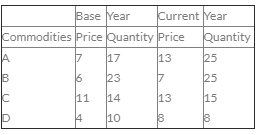The Marshal Edgeworth index number is:

Solution:
QUESTION: 4

Cost of living index numbers are also used to find real wages by the process of:

Solution:
QUESTION: 5

Circular test is satisfied by:

Solution:
QUESTION: 6

The consumer price index for 2006 on the basis of 2005 from the following data is :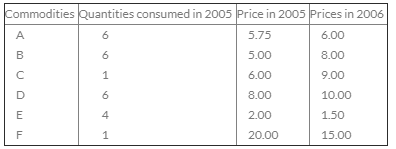Solution:
QUESTION: 7

Net monthly salary of an employee was Rs. 3000 in 1980. The consumer price index number in 1985 is 250 with 1980 as base year. If he has to be rightly compensated, then the Dearness Allowance to be paid to the employee is:

Solution:
QUESTION: 8

From the following data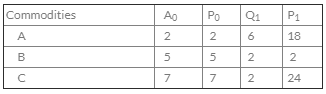Then the fisher quantity index number is

Solution:
QUESTION: 9

The consumer price Index for April 1985 was 125. The food price index was 120 and other items index was 135. The percentage of the total weight of the index is

Solution:
QUESTION: 10

Net monthly salary of an employee was Rs. 3000 in 1980. The consumer price index number in 1985 is 250 with 1980 as base year. If the has to be rightly compensated then, 7th dearness allowances to be paid to the employee is:

Solution:
QUESTION: 11

Net Monthly income of an employee was Rs. 800 in 1980. The consumer price Index number was 160 in 1980. It is rises to 200 in 1984. If he has to be rightly compensated. The additional dearness allowance to be paid to the employee is:

Solution:
QUESTION: 12

Circular test, an index constructed for the year 'x' on the base year 'y' and for the year 'y' on the base year 'z' should yield the same result as an index constructed for 'x' on base year 'z' i.e. I01 x I12 x I20 equal is:

Solution:
QUESTION: 13

Time Reversal Test is represented symbolically is:

Solution:
QUESTION: 14

If the price index for the year, say 1960 be 110.3 and the price index for the year, say 1950 be 98.4, then the purchasing power of money (Rupees) of 1950 will in 1960 is

Solution:
QUESTION: 15

From the following sata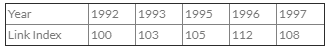(Base 1992 = 100) for the years 1993 - 97. The Construction of chain index is:

Solution:
QUESTION: 16

During a certain period the cost of living index number goes up from 110 to 200 and the salary of a worker is also raised from Rs. 330 to Rs. 500. The worker does not get really gain. Then the real wages decreased by:

Solution:
QUESTION: 17

In 1976 the average price of a commodity was 20% more than in 1975 but 20% less than that in 1974 and more over it was 50% more than that in 1977. The price relatives using 1975 as base year (1975 price relatives = 100) then the reduce date is:

Solution:
QUESTION: 18

The prices of a commodity in the years 1975 and 1980 were 25 and 30 respectively, taking 1975 as base year the price relative is:

Solution:
QUESTION: 19

The total value of retained imports into India in 1960 was Rs. 71.5 million per month. The corresponding total for 1967 was Rs. 87.6 million per month. The index of volume of retained inports in 1967 composed with 1960( =100) was 62.0. The price index for retained inputs for 1967 our 1960 as base is

Solution:
QUESTION: 20

When the cost of Tabacco was increased by 50%, a certain hardened smoker, who maintained his formal scale of consumption, said that the rise had increased his cost of living by 5%. Before the change in price, the percentage of his cost of living was due to buying Tobacco is

Solution:
QUESTION: 21

If ΣP0Q0 = 1360, PnQ0 = 1900, PoQn = 1344, PnQn = 1880 then the Laspeyre Index number is

Solution:
QUESTION: 22

During the certain period the C.L.I. goes up from 110 to 200 and the Salary of a worker is also raised from 330 to 500, then the real terms is

Solution:
QUESTION: 23

The Bowley Price index number is represented in terms of:

Solution:
QUESTION: 24

The price of a commodity increases from Rs. 5 per unit in 1990 to Rs. 7.50 per unit in 1995 and the quantity consumed decreases from 120 units in 1990 to 90 units in 1995. The price and quantity in 1995 are 150% and 75% respectively of the corresponding price and quantity in 1990. Therefore, the product of the price ratio and quantity ratio is:

Solution:
QUESTION: 25

Fisher price index number equal is:

Solution:
QUESTION: 26

Consumer price index number goes up from 110 to 200 and the Salary of a worker is also raised from Rs. 325 to Rs. Therfore , in real terms, to maintain his previous standard of living he should get an additional amount of:

Solution:
QUESTION: 27

From the following data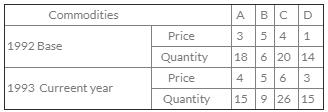The Passche price Index number is:

Solution:
QUESTION: 28

The price of a number of commodities are given below in the current year 1975 and base year 1970.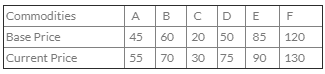For 1975 with base 1970 by the Method of price relatives Geometrical mean, the price index is:

Solution:
QUESTION: 29

From the following data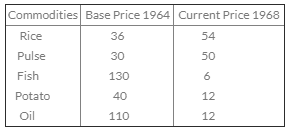The index number by unweighted methods:

Solution:
QUESTION: 30

Cost of living index (C.L.I.) numbers are also to find real wages by the process of

Solution:
QUESTION: 31

From the following data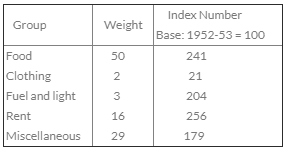The cost of living index numbers is:

Solution:
QUESTION: 32

The prices of a commodity in the year 1975 and 1980 were 25 and 30 respectively taking 1980 as base year the price relative is:

Solution:
QUESTION: 33

From the following data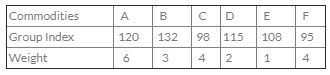The general Index I is given by:

Solution:
QUESTION: 34

From the following data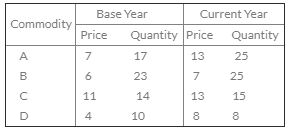The Marshall Edge Worth Index number is:

Solution:
QUESTION: 35

The average price of certain commodities in 1980 was Rs. 60 and the average in 1982 on the basis of 1980 was 100%. 80. The decrease in 1980 with 1982 as base is: using 1982, comment on the above. Statement is:

Solution:
QUESTION: 36

The value index is equal to:

Solution:
QUESTION: 37

Circular Test is not meet by:

Solution:
QUESTION: 38

The Time Reversal Test is not satisfied to:

Solution:
QUESTION: 39

Given below are the data on prices of some consumer goods and the weights attached to the various items Compute price index number for the year 1985 (Base 1984 = 100)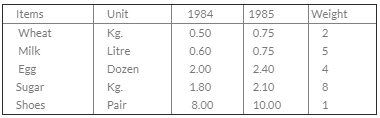Then weighted average of price Relative Index is:

Solution:
QUESTION: 40

If the 1970 index with base 1965 is 200 and 1965 index with base 1960 is 150, the index 1970 on base 1960 will be:

Solution:Use Code STAYHOME200 and get INR 200 additional OFF Use Coupon Code